Main   Article 7Z68 by Richard Pavlicek# Mapping Bridge Deals

Before I explain my mapping process, let’s consider the number of possible bridge deals. It’s an enormous number, but it is easily calculated with combinatorial arithmetic. The formula for the number of combinations of N items taken R at a time is given by:

N!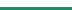R! × (N-R)!

The notation N! (N factorial) means the product of all integers from 1 through N. For example, 6! = 720.

First, let’s use this formula to determine the number of bridge hands that can be dealt to one player. Setting N=52 and R=13, the calculation becomes:

52!13! × 39!

This is the number of possible bridge hands, equal to 635,013,559,600, but we still haven’t constructed a full deal. Now consider the second player. Only 39 cards remain, so the number of combinations is obviously fewer. Setting N=39 produces:

39!13! × 26!

This is the number of possible hands a second player can receive after the first player’s hand is determined, which is equal to 8,122,425,444. For the third player only 26 cards remain, so it becomes:

26!13! × 13!

This equals 10,400,600. Note that there are now only 13 cards left, which perforce go to the fourth player. That is, once you have determined any three hands, you have a unique bridge deal.

The number of possible bridge deals is calculated by multiplying the above three numbers. Note how the factors 39! and 26! neatly cancel out to leave:

52!(13!)4

This equals 53,644,737,765,488,792,839,237,440,000 or 53 octillion if you began to say it. I don’t ever want to see this number again, so from here on I will just call it D (for “Deals” or “Damn, that’s big,” pick one). TopMain

##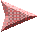Mapping a Number to a Deal

Now suppose you are given a number, ranging from zero to D-1. Intuitively, this should represent one and only one bridge deal, which should be different from the deal produced by any other number. In other words, there should be a one-to-one correspondence between each number and its deal. The first task is to map the number (call it I) to the specific deal it represents.

My algorithm is outlined below. Line 1 initializes the vacant slot count for each player (NESW), the total number of cards (C) and a variable K which tracks the “space” into which all the remaining card distributions must fit. The loop beginning at Line 2 is executed 52 times, once for each card which is given to the player whose counter is decremented.

 1. N=E=S=W=13; C=52; K=D 2. X=K*N/C; If I < X then N=N-1, go to 6 3. I=I-X; X=K*E/C; If I < X then E=E-1, go to 6 4. I=I-X; X=K*S/C; If I < X then S=S-1, go to 6 5. I=I-X; X=K*W/C; W=W-1 6. K=X; C=C-1, loop if not zero to 2

To understand it, consider what happens on the first iteration to place one card, say the spade ace. The first value of K is equal to D and N/C (13/52) is equal to 1/4, so I’m dividing the space into four equal parts:

0------------X-----------X´-----------X´´-----------D

If the number I is less than X, then the spade ace goes to North, and the space from 0 to X must represent all the possible deals where North holds the spade ace. Hence, the remaining 3/4 of the space is discarded (the spade ace would belong to East, South or West) and the next space K is set to X.

If the number I falls in the range: X <= I < X´, then the spade ace goes to East. Now we must discard the space from 0 to X, as well as the space above X´. For ease in calculation, the new space is aligned at zero and the number I is reduced accordingly to keep its relative position. Similar logic applies when I falls in the upper regions, giving the card to South or West.

On subsequent iterations the space is not divided into fourths but into portions that are proportionate to the number of vacant slots left for each player. In each case the size of the portion represents all the possible remaining deals if the current card went to that player. The number I determines which portion is chosen, the card goes to that player, and that portion becomes the new space. When a player runs out of vacant slots the size of his portion calculates to zero.

It is enlightening to note that repeated calculations of the variable X will always produce integers due to the makeup of D. I wanted to exploit this further by calculating K/C at the beginning of each loop (since the ratio is used up to four times) but this interim step can be a fraction that is removed on later multiplication. Therefore, to avoid complications in implementing the algorithm, I multiply first. TopMain

##Mapping a Deal to a Number

Now suppose you want to reverse the process. That is, given a specific deal, determine its number I. This can be done with a similar algorithm:

 1. N=E=S=W=13; C=52; K=D; I=0 2. X=K*N/C; If N card then N=N-1, go to 6 3. I=I+X; X=K*E/C; If E card then E=E-1, go to 6 4. I=I+X; X=K*S/C; If S card then S=S-1, go to 6 5. I=I+X; X=K*W/C; W=W-1 6. K=X; C=C-1, loop if not zero to 2

The number I starts at zero. If the first card (spade ace) belongs to North, I is unchanged; if it belongs to East, I is increased by the space necessary to represent all the deals where North held the spade ace; if it belongs to South, I is increased by North’s and East’s space, etc. In other words, on the first iteration I is set to the base of the quadrant for the player who holds the spade ace, and that quadrant becomes the new space K.

On subsequent iterations the space is apportioned according to the number of slots remaining for each player, and I is increased by the distance to the base of the portion for the player who has that card. Intuitively, this should build a unique number for each deal.

Note that the number I remains at zero if the first 13 cards were held by North, the next 13 by East, and the next 13 by South. Conversely, it reaches the maximum (D-1) if they were held by West, South, and then East. Otherwise, it would fall somewhere in between. TopMain

##Testing the Algorithms

The major difficulty in testing these algorithms is the unwieldy arithmetic, though it is easy to implement by computer. Consider the number D. This translates to 96 binary bits or 12 bytes, which is a convenient size to work with. In hexadecimal (base 16) it is:

AD55 E315 634D DA65 8BF4 9200

What about the order of cards? I order them by rank (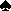A,A,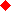A,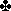A,K,2) but this doesn’t matter as long as it’s the same for both algorithms.

I have implemented these algorithms using my own 96-bit math routines* and tested them many times. One test procedure is to choose a random number and create a deal with the first algorithm, then I use that deal to generate a number with the second algorithm. Do the numbers match? Yes, every time. I have also tested in reverse fashion (deal to number, then number to deal) and the deals matched as well. Therefore, I am convinced the algorithms work.

Of course, one still must rely on theory here because there is no way to test every deal. Imagine this: If you had a million computers, and each computer tested a million deals per second (an amazing performance) it would take over a billion years — 1,701,063,475 to be exact. Mind boggling, no? (OK, OK, it would actually be a little less — I forgot leap years.)*Computer programming has been my main hobby since 1981, the year I got my first computer. I chose to learn assembly language because speed and compactness were so important in the early days — my first computer was a 2 MHz Z80 with only 64K memory and no hard disk! Even to this day I do my programming in assembly language but now it’s a disadvantage, mainly due to its platform dependency on the “x86” family. Today’s fast CPUs with massive memory and storage capacity make software efficiency almost insignificant for most applications, a sad fact that takes much of the art out of programming. Most software now is bloated beyond belief because that makes it easy to put together and quicker to sell, which is really the bottom line after all. It’s not how well you design a program but how fast you can sell it. Sigh.

Acknowledgment: Thanks to Thomas Andrews for his help in correcting flaws in my first attempt to write the number-to-deal algorithm. Thomas has successfully tackled this project using a completely different method. Take a look at his “Impossible Bridge Book” (http://bridge.thomasoandrews.com/impossible). TopMain